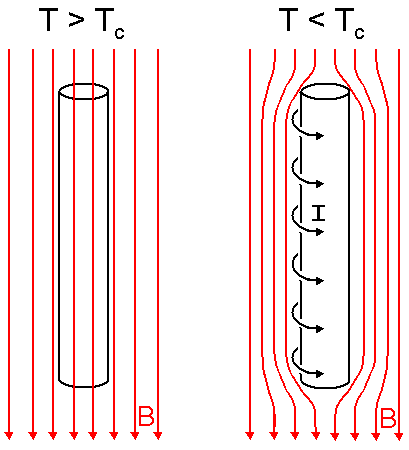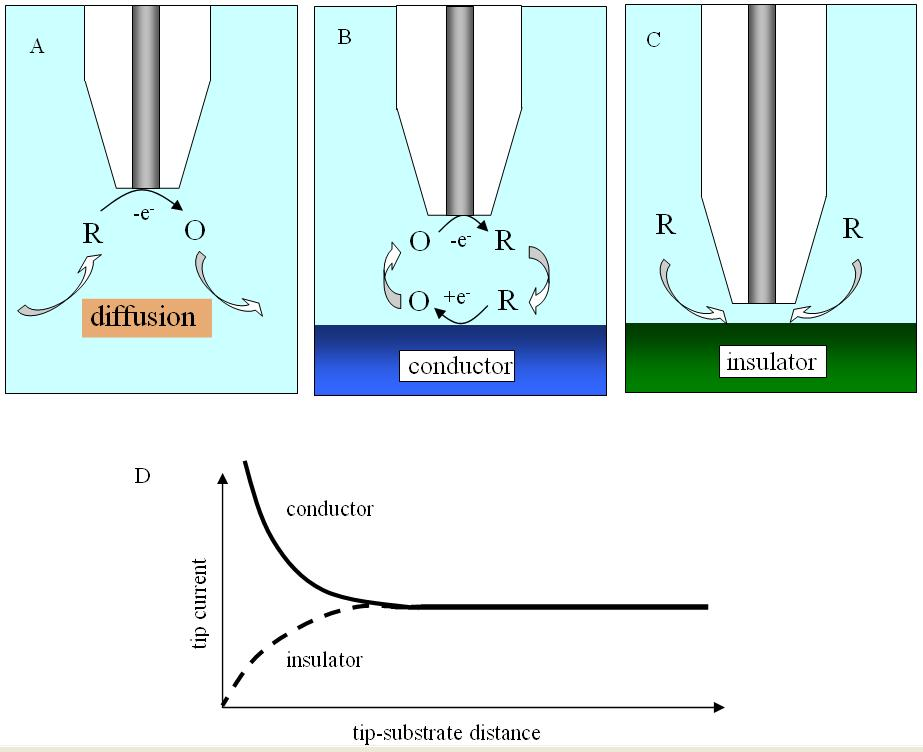Difference Between Superconductor and Perfect Conductor

Super conductors and perfect conductors are two terms which are widely used but often mixed with each other. There are off course a lot of similarities between these two concepts, mainly because both are types of special conductors, but there is a clear delineation as far as their operating principles are concerned.

Superconductors actually exist, but a perfect conductor is a concept, or a scenario, assumed by the physicists to simulate properly (to make the calculations easier). In actuality, there is nothing like a perfect conductor, much in the same way like there is nothing like an ideal gas.

Perfect conductors can exist at any temperature, but the superconductors only exist below the critical temperature of the material.

The conductance of any material depends upon its resistivity. Greater the resistivity of the material, lower will be its conductance and vice versa. Resistivity, which is basically resistance posed to the flow of current and heat, is one of the basic properties of any material, and the same applies to various elements and materials which are used in the field of electronics and electricity generation and propagation (here the attribute of interest is current). The resistance of any material can also be defined in terms of voltage. When the current flows through a conductor, its resistance poses a hindrance to the flow, which causes the voltage to differ at each end of the conductor material. The voltage difference is an apt indicator of the resistance of the material, which can be defined as a ration of voltage differential to the current passed.

Instructions

• 1

Superconductor

Superconductivity is a phenomenon in which the material has zero resistivity when it is subjected to certain characteristic temperature. This phenomenon is only observed in certain materials. In terms of theory, if the material is a superconductor, it cannot support a magnetic field inside it. This state of material only exists below the critical temperature, reaching which if often quite difficult – less than 10 Kelvin. However, once the material has achieved this state, its resistance falls abruptly to zero. More recently, some high temperature superconductors have been discovered, which can attain the zero resistance state at temperatures as high as 130 K.

- Image Courtesy: supertightstuff.com• 2

Perfect Conductor

In reality, the resistance of a conductor depends on the availability of the free electrons, which are abundant in elements like coppers, iron etc. As discussed earlier, in order to attain superconductivity, or high conductivity for that matter, a lot of external factors are required, the biggest one being the sub critical temperatures. That is not the case in most of the simulation scenarios. So, in order to simplify the calculations, an assumption of perfect conductor is made.

- Image Courtesy: electrochem.cwru.edu•